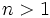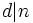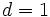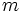# Subwiki:Property definition

On certain subject wikis following a paradigm of property-theoretic organization, many of the terms being defined are properties. This article discusses the forma of definition for properties.

## What is a property?

A property over a collection of objects is something that every object in the collection either satisfies or does not satisfy. Thus, the collection can be partitioned into two subcollections: those objects that satisfy the property, and those objects that do not satisfy the property.

The collection of objects over which a property can be evaluated is termed the context space of the property. For instance, the property of being prime can be evaluated over natural numbers; in this case, the context space for the property of being prime is natural number. Primality is thus a property of natural numbers.

### Word forms for the property

• Adjective: For instance prime is an adjective describing the property of being prime. The adjective modifies the noun for the context space, so a number that is prime is termed a prime number.
• Instance-noun: For instance, perfect square is a noun describing the property of being a perfect square. Here, the noun describes an object satisfying the property.
• Property noun: For instance, primality is a property noun describing the property of being prime.

## Goals of the property definition

### What the property definition should provide

The definition should make clear:

• What the context space is, over which the property is being evaluated.
• How, given an object in the context space, one can determine whether or not it satisfies the property. The criteria should be necessary and sufficient: they should reject everything that does not satisfy the property, and accept everything that does satisfy the property.
• What other properties the given property builds upon in its definition.

## Presenting the property definition

### The symbol-free definition

A symbol-free definition is a definition free of symbols, and using natural language as far as possible. Symbol-free definitions often provide more useful and memorable formulations of properties, highlighting more clearly the relation with existing properties. Here are the typical formats of a symbol-free definition:

1. A (context space object) is (said to be)/(termed)/(called) (property name) if ....
• (adjectival example) A natural number is termed prime if it is greater than one and the only natural numbers dividing it are one and the number itself.
• (instance-noun example) A natural number is termed a perfect square if it is the square of a natural number.
• (property noun example) A natural number is said to satisfy the property of primality if it is greater than one and on the only natural numbers dividing it are one and the number itself.
2. A (property name) is a (context space object) satisfying ...
• (adjectival example) A prime number is a natural number greater than one for which the only natural numbers dividing it are one and the number itself.
• (instance-noun example) A perfect square is a natural number that can be expressed as the square of a natural number.
• (property noun example) Primality is the property of a natural number being greater than one and not having any prime divisors other than one and the number itself.

### Definition with symbols

A definition with symbols is a definition that sets explicit symbols for the object for which we're providing the criterion. These symbols are used in the definition, in place of pronouns and other natural language constructs. For instance:

1. A (context space object) (symbol introduced) is (said to be)/(termed)/(called) (property name) if ....
• (adjectival example) A natural numberis termed prime ifand for any natural number,if and only ifor.
• (instance-noun example) A natural numberis termed a perfect square if there exists a natural numbersuch that.
• (property noun example) A natural numberis said to satisfy the property of primality ifand for any natural number,if and only ifor.

### Multiple equivalent definitions

In situations where there are multiple equivalent definitions, the definitions are provided as an enumerated or bulleted list, with the context space-setting happening before the enumeration. The enumeration thus looks like:

A (context space object) is (said to be)/(termed)/(called) (property name) if it satisfies the following equivalent conditions: (followed by an enumeration).